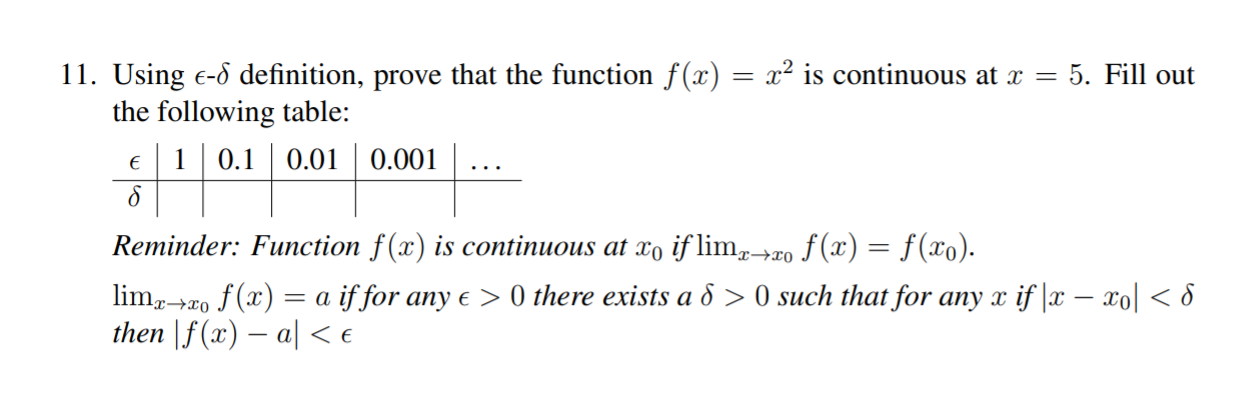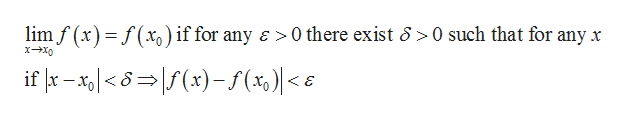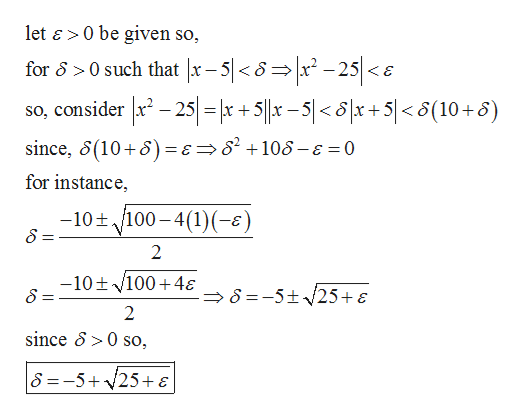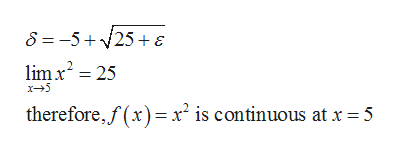# c is continuous at x =11. Using E-8 definition, prove that the function f(x)the following table:e 0.10.015. Fill out0.001Reminder: Function f(x) is continuous at xo if limzo f(x) = f(x0).limro f(x)a iffor any e > 0 there exists a 6 > 0 such that for any x if x - xol

Question
1 views

Good afternoon. I have a midterm coming up, and my professor has given us a few definitions I have never seen before. I cannot seem to make sense of what she is doing, and barely can understand what she is asking for, even when she steps us through them. I was wondering if I could get a plain-english description of what she is looking for, and how to do, the attached question. Thanks!help_outlineImage Transcriptionclosec is continuous at x = 11. Using E-8 definition, prove that the function f(x) the following table: e 0.10.01 5. Fill out 0.001 Reminder: Function f(x) is continuous at xo if limzo f(x) = f(x0). limro f(x)a iffor any e > 0 there exists a 6 > 0 such that for any x if x - xol<8 then \f(x) a< e fullscreen
check_circle

Step 1

According to the given information it is needed to prove that f(x) is continuous at x=a where

f(x) = x2 and a=5

To prove the following use the definition:help_outlineImage Transcriptioncloselim f (x)f(x) if for any a 0 there exist 6 0 such that for any x if -x x)-f(%)| < e fullscreen
Step 2

Now,help_outlineImage Transcriptioncloselet & 0 be given so, for 8>0 such that x-5|<8= x -25 0 so, S =-5+25+ E fullscreen
Step 3

So, for...help_outlineImage Transcriptionclose8 =-5+25+ E lim x2 25 x5 therefore,f(x) x is continuous at x =5 fullscreen

### Want to see the full answer?

See Solution

#### Want to see this answer and more?

Solutions are written by subject experts who are available 24/7. Questions are typically answered within 1 hour.*

See Solution
*Response times may vary by subject and question.
Tagged in

### Math